# Software for Particle Size Distribution Analysis in Photon Correlation Spectroscopy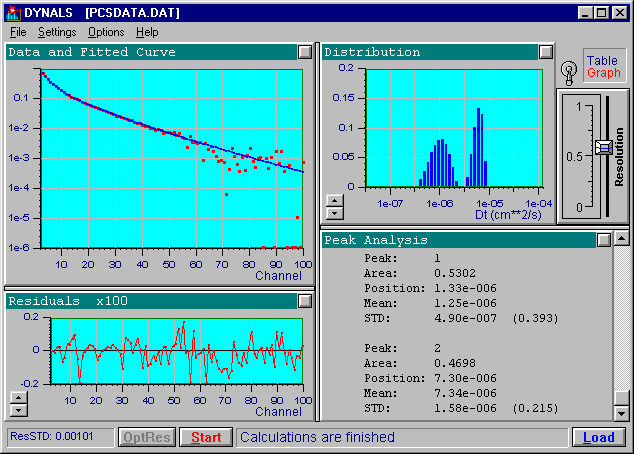This document was written by Dr. Alexander A Goldin (Alango Ltd.)

This document describes the sophisticated DYNALS software and its underlying advanced algorithms for Distribution Analysis of Photon Correlation Spectroscopy (PCS). Its purpose is to help DYNALS users understand and "feel" the problem of Particle Size Distribution Analysis in PCS. It also provides necessary information regarding the algorithms that have been developed by the author over a number of years.

The author is an applied mathematician, not an expert in Photon Correlation Spectroscopy. Therefore, rather than present information about PCS, this document outlines the mathematical aspects of the "ill-posed problem" at hand and discusses a possible means to cure it. An overview of the algorithms implemented in DYNALS is provided, along with a description of how the algorithm's parameters may be changed through the DYNALS graphical interface. While using DYNALS, you will generally not need to alter computational parameters. However, this document includes recommendations regarding how and when these parameters may be altered to extract the maximum amount of information from your experimental data.

This document is neither a scientific paper nor a software manual. It is not rigid in the manner of a scientific paper and contains many practical tips and advices based on the author's personal experience. Unlike a software manual, it does not provide detailed step-by-step explanations of the DYNALS procedures, menu items, data formats, etc. This kind of information may generally be found in the DYNALS on-line help.

This document was last updated in March 2002

Appendix A. Practicing DYNALS
A.1 Understanding the term "ill-posed problem"
A.2 Understanding the resolution and its dependencies

Appendix B. Tagged Data Format (TDF)

## 1. Introduction to the Problem of Particle Size Distribution Analysis in PCS

Photon Correlation Spectroscopy is an indirect measurement method. The measured quantity (the auto-correlation of fluctuations of the scattered light intensity) must undergo further processing to extract the quantity of interest (e.g. the particle size distribution or the diffusion coefficient distribution). In the simple case of a mono-dispersed solution (spherical particles of the same size) and when some other physical conditions are met, the normalized intensity correlation function is described by an exponential plus a unit constant: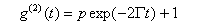The coefficient G is related to the physical properties of the particles and the experimental conditions by the following expressions: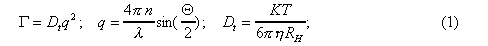where

G is the decay rate
q is the scattering vector
DT is the translation diffusion coefficient
n is the refraction index
l is the scattered light wave length
Q is the scattering angle
T is the absolute temperature of the scattering solution
K is Boltzman's constant
h is the solvent viscosity

In case of a poly-dispersed solution where the particles are not identical, the auto-correlation function of the scattered light intensity is described by the following pair of equations: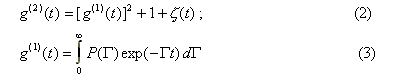Disregarding the influence of inevitable experimental noise x(t) (discussed later), equation (2) allows us to compute the first order field correlation function g(1)(t) from the second order intensity auto-correlation function g(2)(t) accumulated by the correlator during the experiment.

DYNALS' treatment of the integral equation (3) will be the main subject of concern in the remainder of this document. This integral equation actually describes the relationship between the experimental data obtained during the experiment and the physical properties of the solution under study. If the decay rate distribution R(G) is known, then the Diffusion Coefficient Distribution or Particle Size Distribution can be easily computed using the equations (1). Sometimes the physical model may be more complex (e.g. if the particles are not spherical), in which case equations (1) must be replaced with something more complicated. The integral equation (3) forms the basis for data processing in Photon Correlation Spectroscopy.

This kind of equation with respect to R(G) is known as a "Fredholm integral equation of the first kind" and its solution is known to be an "ill-posed mathematical problem." Practically, this means that if the function g(1)(t) (experimental data) is known with even a minute error, the exact solution of the problem (the distribution R(G)) may not exist, or may be altogether different from the actual solution. On the other hand, the approximate solution is never unique. Within the experimental data error there exist an infinite number of entirely different distributions that fit the experimental data equally well. This does not mean that there is no reason to improve the accuracy of the experiment. The experimental error and the structure of the correlator (linear, logarithmic, etc.) define the class of distributions which may be used as candidates for the solution. The better the data, the smaller the number of distributions that fall within that class, thereby leaving fewer alternatives for the algorithm used to solve the problem.

Another method of reducing the number of possible solutions is to look only for non-negative solutions. Since a distribution can never be negative (i.e. the number of particles cannot be negative), this constraint is always valid and its use is always justified. Looking for non-negative solutions dramatically reduces the class of possible solutions and may sometimes even cause the problem to be stable. Generally, however, this is not sufficient. The class of non-negative distributions which fit the experimental data is still too wide to pick one of them at random and assume that it is close enough to the actual distribution. An additional strategy must be used to choose one particular distribution which will satisfy our intuitive notion about the physical measurements. Thus we must have some criterion that will allow us to express our preferences.

Generally, we would like to pick "the smoothest" distribution from the class of possible candidates. The difference between the various algorithms and their implementations is based on how this additional criterion is formulated and realized. In DYNALS, "the smoothest" distribution is quantified as the distribution with the smallest amount of energy, or for mathematicians, as the distribution of the minimal L2 norm.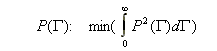The different approaches to the problem are discussed in the ensuing sections of this document.

If you are not familiar with the problem, now is a good time to try out the DYNALS program and the included synthetic data sets. This will give you a better feel for the problem and more importantly, a deeper understanding of DYNALS and what you can and cannot expect from the program. It is very important to play with synthetic data as opposed to real data. In this way, you become familiar with the kind of distribution achieved in the best possible scenario where no noise is present in the data. You can observe how a higher noise level broadens the class of possible solutions and how it influences the resolution achieved. This will also help you attain the practical skills necessary to work with DYNALS in your daily work. Initially, you are advised to follow the instructions described in "Understanding the term "ill-posed problem" in Appendix A.

## 2. Using   DYNALS

This section explains how simple it is to work with DYNALS. DYNALS processes your experimental data and automatically gives you the best possible distribution. Just point and click; the distribution is computed, the results are analyzed, the analysis is stored, and all important information is printed.

The DYNALS on-line help, along with your own intuition, should be sufficient to help you begin the processing and enable you to obtain initial results. This document will help you interpret the results and make sure that information from the experimental data has been properly utilized.

DYNALS is under continuous development. Therefore, the operational instructions provided in this section may not be up-to-date. These instructions should provide you with tips and hints regarding the program's possibilities. Please refer to the on-line help for the exact names of menu items, entry fields and other details.

Loading data is generally the first thing you do after you have started DYNALS. Simply select File > Load data from the menu bar or click the Load button on the Status Bar, and select the desired file. You should see the data displayed in the upper left graphical window. You can set the Y axis to use either the linear or logarithmic scale, and the X axis to correspond to channel numbers or time delay.

At the present time, DYNALS supports two data formats:

• Files with the extension .DAT have a very simple ASCII format. This file contains a normalized second order correlation function measured by the correlator. Every line of the file corresponds to one particular channel and consists of two numbers: the corresponding time delay in seconds and the value of the correlation function. Since this file does not include the physical parameters of the experiment, relevant parameters that are different from the ones used for the previous processing, should be entered manually. Examples of such files are installed during the DYNALS installation procedure.
• Files with the extension .TDF (Tagged Data Format) are ASCII files whose structure is similar to the structure of Windows .INI files. These files may contain different information including the correlation function itself and the parameters of the experiment. Each category of information is stored in a specific section of the file and is provided with its own tag. Therefore, reading the procedure distinguishes between the different types of information and deciphers them correctly. The structure of .TDF files, (i.e. the section and tag names) recognized by DYNALS are described in Appendix B. Examples of TDF files are installed during the DYNALS installation procedure.
Other well-defined formats may be supported in the future. Please contact the authors if you have any suggestions.

### 2.2 Setting the Physical Parameters (optional)

To obtain correct values along the axis of the diffusion coefficient or the hydrodynamic radius axis, you should set suitable values for the physical parameters of the experiment. If the physical parameters are the same as they were for the previous experiment, or they were provided with the data (e.g. in a TDF file), you may skip this section. To set the correct values, select Settings > Processing parameters from the menu bar and enter the desired values. In the current version of DYNALS, the processing parameters are located in the same dialog as the physical parameters. Set the desired processing parameters as described in the next paragraph, or just click the OK button to begin the computations.

### 2.3 Setting the Processing Parameters (optional)

In general, the processing parameters can be set once and left as is. There is no single parameter which defines the stabilization of the problem, rather it is computed automatically and may be altered afterwards without entering the dialog. The parameters set before initiating the processing, define the following:
• The kind of distribution you wish to obtain.

• Available options are: decay times, decay rates, diffusion coefficients and hydrodynamic radii.
• The range of channels for which the fitting takes place.

• Sometimes you may know in advance that some of the correlator channels contain incorrect information which will corrupt the results and prevent a good fit. If such channels are at the beginning or at the end of the correlation function, you can exclude them from consideration by entering the numbers of the first and the last channels of the range you wish to process. Entering --1 as the last channel means "until the last channel".
• The number of discrete intervals in the computed distribution.

• If the number of intervals is high enough, it will not define the actual resolution between the distribution peaks, nor will it define the stabilization of the problem. The resolution is defined automatically and is dependent upon the noise, the correlator structure and the distribution itself. The problem of resolution is discussed later in this document. It is recommended that the number of intervals be set as greater than 50 so the distribution looks continuous and does not impose constraints on the optimization procedure. Using this parameter you can also emulate the method of histogram . Choose a small number of intervals (10-15) and set the maximal resolution after the processing is finished. This corresponds to the situation when the stabilization is accomplished by directly reducing the problem dimension and not by using a regularization procedure. You can easily see that this is an inferior approach.
• The distribution domain.

• This parameter defines the interval on the axis of the physical units where the distribution is computed. If the beginning of the interval (value in the From field ) is larger than the end of the interval (value in the To field), then the default values are used. These values are large enough to restore both the slowest and the fastest exponential components that can be restored. On the one hand exponentials which decay too fast cannot be detected since they vanish before the first channel of the correlator. On the other hand exponentials which decay very slowly appear as a constant and cannot be distinguished from each other. Thus there exists a finite range defined by the structure of the correlator and the sampling time, in which the distribution may be restored. Unless you want to experiment or make comparisons with other software, we recommend using the default values.

### 2.4 Getting Results

If you change one of the physical or processing parameters, the distribution is automatically computed as soon as you leave the dialog. If you just loaded new data, click the Start button to activate computations. No matter how the processing was initiated, the progress indicator on the status bar shows how much work has already been done.

After the processing is completed, the DYNALS window appears similar to the one at the beginning of this document.

In the upper left part of the screen you will see the experimental data together with the fitted curve corresponding to the computed distribution. The lower left part of the screen shows the residual curve, which is the difference between the experimental data and the fitted curve. The residual curve is very important for estimating the quality of fit. For a good fit the residual curve should look random. If it does not look random either the data is 'underfitted', or there is a systematic error present in the data. In both cases the results are probably invalid.

The computed distribution is displayed in the upper right part of the screen and may be displayed as a graph or table depending on the position of the selection handle at the right. The distribution is computed using a logarithmic scale along the horizontal axis. The height of each column indicates the relative distribution area under this column which, due to the logarithmic scale, is not the same as its amplitude. The area under the entire histogram is normalized so that it is equal to one.

The lower right corner displays an analysis of the computed distribution. This window presents parameters of the entire distribution, its peaks and subpeaks. Note that the mean and the standard deviation of the entire distribution correspond to the values being computed and the method of cumulants. However, these values are generally computed more accurately by the distribution analysis.

### 2.5 Resolution Slider Value and Other Processing Parameters

When the distribution is computed, the resolution slider in the upper right corner of the DYNALS window is enabled and the OptRes button on the status bar remains disabled. This means that the distribution displayed in the upper right window is computed using the optimal resolution. The terms 'resolution', 'optimal resolution' and 'maximal resolution' are important to your understanding of DYNALS and are, therefore, discussed here in greater detail.

You may use DYNALS without actually knowing what these terms mean. If this is the case, use the default distribution domain parameters and the number of distribution intervals larger than 50. If the residual values (bottom left window) look random, don't try to 'improve' the distribution by altering the resolution slider -- the distribution computed with the optimal resolution contains all the information that can be extracted from the experimental data. If you have absolutely reliable a priori information that your distribution consists of a small number (1 - 4) of very narrow peaks, you may try to set the maximum possible resolution by shifting the resolution slider to its upper position. This may dramatically improve the resolution due to the impact of the additional information you provided. Note, though, that if your information is incorrect, you will obtain a sharp but erroneous distribution. If the residual values do not look random then either you have a problem with your data (most probable), or the algorithm made a faulty estimation of the experimental noise. The following paragraphs explain how to verify this aspect of the distribution.

As mentioned above, the problem of computing the Particle Size Distribution from PCS data (or any related distribution) is an unstable, "ill-posed mathematical problem." The solution is not unique and generally should not be the one that provides the best possible fit for the experimental data in accordance with equation (2). Instead the solution should provide a reasonable fit which is stable enough. 'Reasonable fit' usually means 'up to the accuracy of experimental data. 'Stable enough means 'the one that does not change drastically with small changes in the experimental data. For example, we would expect to get similar distributions from several experiments made on the same sample although the data are slightly different due to inevitable experimental noise. When the random error in the experimental data increases, stability is improved by choosing a smoother distribution. In other words, the resolution decreases as the error increases.

The stability is always improved by reducing the number of degrees of freedom in the solution. The simplest way to reduce the number of degrees of freedom is to divide the distribution domain into a smaller number of intervals. For example, if you set the number of logarithmically spaced intervals as 10, this, together with non-negativity constraints on the solution, makes the problem mathematically stable. This kind of stabilization, sometimes called "naive" , constitutes the essence of the method of histogram . Unfortunately, the approximation of the distribution of interest with only 10-12 intervals is too irregular. It thus prohibits the reconstruction of details which can be attained using more sophisticated algorithms. You may easily check this claim by setting the number of distribution intervals in DYNALS to 10 and shifting the resolution slider to its maximal value, thus effectively performing the method of histogram.

If the number of distribution intervals is large enough (recommended value is 50 or larger), the resolution slider position shows the trade-off between the fit of experimental data and the maximum attainable resolution. It should be understood that resolution along the G axis is not constant. It varies for different values of G and depends on the accuracy of experimental data, the structure of the correlator and on the distribution itself. For example, with good authentic data, it is possible to resolve two delta functions d(G- G1), d(G- G2) (two peaks) with G2 / G1 =3 when they are in optimal position on the G axis (about the middle of the default distribution domain) Footnote 2 . The same resolution (3:1) is not possible if delta functions are located closer to the DYNALS default domain ends or if additional components are present in the distribution. You may learn more about the resolution and its dependence on the above factors by practicing the exercises "Understanding the resolution and its dependencies" in Appendix A.

If the resolution slider value is too low, the distribution will not have enough detail to provide a good fit for the experimental data. If the value of the resolution slider is too high, the data will be "overfitted", where the details of the distribution may not be reliable due to the noise in the experimental data. The optimum value is in between, so that the resolution slider value is the smallest one that provides a good fit. The stabilization algorithm implemented in DYNALS automatically finds and sets this optimum value.

In general, you will not need to alter the optimal resolution slider value once it has been set after new experimental data has been processed for the first time. Whether your data are real or simulated with a reasonable noise, you will always get a value between 0 and 1 for resolution slider.

There are three cases where you might want to alter the optimal resolution value:

• The first case, as described above, occurs when you know a priori that the distribution of interest consists of a small number (1-4) of very narrow peaks. In this case you can shift the resolution slider to the maximum value and get the accurate positions and amplitudes of these peaks. Utilizing the additional information, you may obtain a resolution that is much better than the optimal one. You should exercise caution in this case, since the computed distribution will always consist of narrow peaks even if the true distribution is continuous and wide. This is the consequence of using incorrect information. You can see these effects by using the maximal resolution while doing the exercises in Appendix A.
• The second case is where you doubt that the found value is really optimal. The optimal value should be the smallest one that makes the residual graph to look random. If it looks random, try to reduce the value until the shape of the distribution changes significantly. If you see that the distribution is noticeably different but the residuals and their standard deviation are still the same, then the algorithm has underestimated the error. (This case is rare, but theoretically possible.)
• The third case is where the opposite situation occurs, and there is a significant, not random (systematic) component in the residuals. In that case, try to increase the resolution slider value. If it cures the residuals, the algorithm overestimated the real error. If it does not help, the experimental data does contain some systematic error. Distributions obtained from such data may be very different from the actual ones since the algorithm has no way to differentiate between the systematic error and the data.
This document has already discussed two of the three parameters that influence the shape of the computed distribution. The last parameter is the domain where the distribution is computed. The distribution domain is defined by its lowest and highest values, and the distribution is considered to be zero outside the specified interval. This, of course, may not be the real case, but our ability to reconstruct the distribution outside certain limits is very limited. Exponentials that correspond to small particles and decay rapidly, may vanish too quickly to leave any trace even in the first correlator channel. Consequently, they cannot be detected and in accordance with the parsimony principle should be considered non-existent. On the other hand, exponentials that correspond to relatively large particles, decay so slowly that they cannot be distinguished from a constant. This constant will appear as a wide peak in the corresponding part of the distribution. No resolution or localization is possible in that area, only the detection of the distribution's presence Footnote 1 . The default distribution domain used in DYNALS is wide enough to accommodate the entire range possible for reliable reconstruction. If you think that it is better to use a wider domain, feel free to enlarge it. A wider range will not be detrimental, provided that the number of intervals is large enough to allow DYNALS to reconstruct the details of the distribution. The author does not recommend reducing the domain. It cannot improve the resolution, and may distort the distribution shape if significant portion of the distribution is left out.

## 3. More Mathematics (optional section)

This section is optional and provides a deeper understanding of mathematical difficulties related to extracting the Particle Size Distribution or related distribution from PCS experimental data. This understanding is not essential in order to work with DYNALS. This chapter is designed to free the user from the mathematics and simultaneously provide enough flexibility to check and control the computations where there may be some doubt regarding the computed results; it is for those cautious users who want a thorough understanding of this rather complicated problem of applied mathematics and the relationship between its mathematical properties and the results obtained using DYNALS or other software. The author wishes to state that when using DYNALS, understanding the math will not improve the results, just as a thorough understanding of the combustion engine does not generally help you drive your car. Both DYNALS and a modern car are sophisticated enough to help you reach your intended destination without requiring a deep understanding of what is going on inside.

Let us begin with the solution to the integral equation (3). The document will present a numerical analysis of the problem (3) in the discretized form and explain why this problem stands as one of the most severe inverse problems in applied mathematics. This section investigates the corresponding system of linear equations and how the properties of the matrix are reflected in the solution. The traditional approaches to the problem are discussed, both the ones which are specific to the equation (3) and the ones having the equation (3) as a particular case. An introduction to the algorithms implemented in DYNALS is then presented, in order to give you an understanding of why these algorithms are superior to the traditional ones. The section also discusses possible improvements which may be implemented in future versions of DYNALS. At the end of this section, the author briefly reviews the complications caused by using the second order correlation function g(2)(t) accumulated by the correlator to compute the field correlation function g(1)(t) used in the equation (3).

### 3.1 Discretization of the Problem and the Maximal Reconstruction Range

This section abandons the continuous representation of the problem defined by equation (3) and begins working with more practical, discrete representations.

First of all, the correlation function g(1)(t) is known only on a limited set of points {ti}, i=1,M , where M is the number of correlator channels. (The problem of computing g(1)(t) from g(2)(t) is discussed later). The set is defined by the particular correlator structure in use. This structure may or may not be uniform. This discretization of equation (3) leads us to the following system of equations: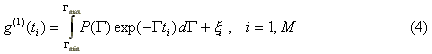where xi are the noise values, and the integration boundaries of equation (3) are replaced with [Gmin,Gmax]. The replacement of the integration boundaries reflects the loss of information caused by the finite correlation time ranges covered by the correlator channels. Indeed, the small values of G correspond to slow decays of the correlation function so that Gmin can always be chosen small enough, such that if G< Gmin then exp(-Gti) is indistinguishable from a straight line (and hence from each other) due to the errors xi . On the other hand, large values of G correspond to fast decays of the correlation function. Due to a finite value of t1 , there always exists a value Gmax such that if G> Gmax then exp(-Gti) falls below the noise level before the first channel. The idea is illustrated by the following figure: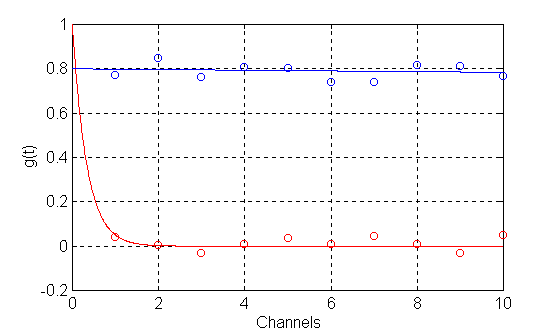Figure 1. Blue solid line - exp(-Gti) for G< Gmin , blue circles - its measured values;
red solid line - exp(-Gti) for G> Gmax , red circles - its measured values

It is clear that while using only the measured values {g(1)(ti)}, i=1,10 it is impossible to distinguish the blue curve from the constant and the red curve from zero. In DYNALS, the default range for G is set as [Gmin=0.1/tM , Gmax=5/t1] so that it is wide enough to cover the maximal possible range for even the smallest possible noise in the correlator channels.

Having the set of equations (4), the next step is to find a parametric representation for P(G) that is both non-restrictive and allows the use of an effective numerical algorithm. Various representations have been proposed which lead to different reconstruction methods. For example, using the first cumulants of P(G) leads to the method of cumulants [3,4]. Using a parametric distribution of particular shape (or their superposition) with unknown parameters leads to a nonlinear fitting procedure. Examples include several delta functions for multi-exponential fit, Gaussians , Pirson distribution , log normal distribution  and some others. Specifying the distribution as a number of a priori spaced delta functions leads to the exponential sampling method  when the number of delta functions is small, or to regularization methods  like the one used in CONTIN [8,9] when this number is large. When the distribution is approximated by a relatively small number of histogram columns (10-12) with specified boundaries, the method is called "the method of histogram" [10,11]. A short survey of the methods appears in Section 3.3.

In DYNALS, as in the method of histograms, the distribution in the range [Gmin, Gmax] is also approximated by a number of rectangular columns. The columns are adjacent to each other, where the column boundaries are spaced using the logarithmic scale substantiated by the behavior of the eigen functions of equation (3) [12,13]. Denoting the boundaries of N columns as { Gi } where i=0..N, Go=Gmin , GN=Gmax leads to the system of M linear equations with N unknown coefficients {xi}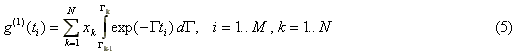or in a compact form: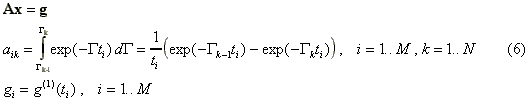The difference between the discretization in DYNALS and in the method of histogram is in the number of histogram columns used to represent the distribution. In the method of histogram, the stabilization of the problem is achieved by reducing the number of columns N to about 10-15. Using a small number of columns to approximate the distribution of interest leads to a very coarse distribution with pure resolution and appearance. In DYNALS, the stabilization is achieved by using the developed, proprietary method for solving the above system of equations while constraining the solution to remain non-negative. Additionally, special scaling for columns of the system matrix A, together with the solution of the minimal norm ||x|| leads to the computation of the distribution P(G) having the smallest L2 norm (or energy in physical terms) that is: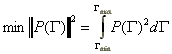Numerous computer experiments have shown this property to be very useful for proper reconstruction of the distribution of interest.

Another important factor that must be taken into account is that errors in the elements of the vector g are unequal (see Section 3.5). Numerically it is accomplished by replacing the original system (6) with the weighted system Awx=gw , where Aw=WA, gw=Wg and W is the diagonal matrix with elements wii =1/si  inversely proportional to the standard deviation of the corresponding values gi. To keep the notations simple, the superscripts denoting weighting will be omitted in the following sections. However, it should be noted that the weighted system of linear equations is always used in the actual computations made by DYNALS.

### 3.2 Numerical Analysis of the Problem

Now that the system of linear equations (6) has been associated with the original integral equation (3), we will analyze it in order to understand why this problem is thought to be one of the toughest problems in applied mathematics.

We will use Singular Value Decomposition (SVD) as the main analyzing tool. The singular value decomposition of a rectangular, mxn matrix A is defined as A=USVT , where U and V are orthogonal matrices and S is a diagonal matrix  with non-negative elements. An orthogonal matrix is a matrix whose transposed matrix is equal to its inverse i.e. UUT= UTU=I where I is the identity matrix. They have the remarkable property that ||Ux||=||UTx||=||x|| i.e. multiplying a vector by an orthogonal matrix does not change the vector's length. This property is very important since it allows us to characterize such matrices as "good" matrices; if we have a system of linear equations Ux=g, the solution of the system is trivial x=U-1g= UTg (see the definition of orthogonal matrix above). When =g'+dg , where dg designates an error component in the right hand side, the solution vector is composed from two components x=x'+dx=UTg+UTdg. When ||x||=|| UTg||=||g|| and ||dx||=||UTdg||=||dg|| , it follows that ||dx||/||x||=||db||/||b|| is the relative error in the solution and is always equal to the relative error in the data. A better solution is unavailable without additional information.

Unfortunately, the properties of the system matrix A are defined by the diagonal matrix S, which in our case is a problematic matrix. Let us make some transformations of the original system of linear equations (6) to see what is so problematic in this very simple, diagonal matrix S. Replacing the matrix A with its singular decomposition gives us the system USVTx=g. Multiplying the right side of this system by UT results in SVTx=UTg (see the definition of the orthogonal matrix). By making simple changes in the variables p=VTx, f=UTg we get a new system of equations Sp=f .

Recalling that the matrix S is diagonal, the solution of this system may seem to be trivial p=S-1f=[f1/s1,f2/s2,.. fN/sN]T . Unfortunately, our case is not that simple. If the right side of the initial equation is known with an error dg, then f=f'+df where df=UTdg, and in accordance with the property of orthogonal matrices above, ||df||=||db||. Due to the error dg, using the same property, the error in the solution p is dp= S-1df and ||dp||=||dx||. It is now apparent that the sensitivity of the solution to errors in the data is defined exclusively by the matrix S. The diagonal elements of matrix S are called the singular numbers of matrix A, and their properties resemble the properties of the singular numbers of the functional operator (3). It may be shown that: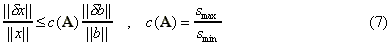where c(A) is known as the "matrix condition number". This equation states that the relative error in the solution vector is proportional to the relative error in the data vector and the condition number of the system matrix. When the ratio of the two extreme singular numbers is small, the matrix (and the problem) is termed "well-conditioned". The best possible case is, obviously, c(A)=1 which corresponds to orthogonal matrixes. When the ratio of the two extreme numbers is large, the matrix (and the system of linear equations) is termed "ill-conditioned" which resembles the term "ill-posed" used to describe the parent, continuous integral equation (3). When the minimal singular number is zero (they are always non-negative), the matrix and the system of equations are called "singular".

In our case, the singular numbers of the system matrix A decay to zero very quickly, thus complicating the problem. The following figure illustrates the decay of singular numbers for three cases: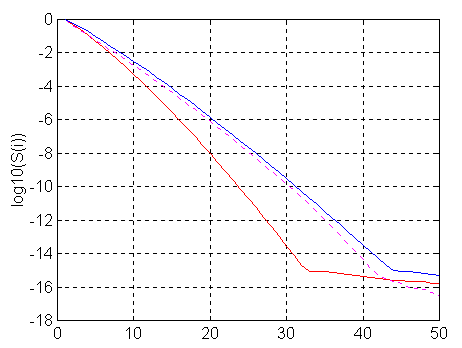Figure 2. red line - logarithms of singular numbers for uniform structure with M=100
blue line - logarithms of singular numbers for uniform structure with M=500
magenta dashed line - logarithms of singular numbers for logarithmic structure with M=50

The leftmost, red solid line shows the logarithm of the singular numbers for uniform distributed data points {ti=Dt*i}, i=1, M, where M=100, Dt=1 and [Gmin=0.002, Gmax=5]. The blue line corresponds to the same conditions but for M=500. Enlarging the number of correlator channels alleviates the problem, however the improvement is not dramatic and does not transform the problem into a "well-behaved" one. It does extend the possible reconstruction range towards smaller gammas in proportion to the number of channels (to Gmin=0.0004 in this case). The same effect may be achieved using a much smaller number of logarithmically distributed channels covering the same range (1 - 500). The magenta dashed line shows the singular numbers for M=50 and { ti= ti-1+max( floor(exp(i*q)), 1) }, i=2, M , t1=1 and the constant q is chosen for tM=500.

The singular numbers decay so fast that they drop below the single precision computer arithmetic somewhere around the 20th singular number and below the level 10-3 corresponding to a suitable accuracy in the correlation function just after the tenth number. If we recall the relation (7), we understand why the maximal number of histogram columns cannot be larger than 12. The relative errors in the histogram's column heights become so large that they are unacceptable.

### 3.3 Traditional Approaches to the Problem

There is no way to make an ill-conditioned (ill-posed) problem behave well other than reducing the number of its degrees of freedom. If the function of interest cannot use an arbitrary shape to fit the experimental data, it must obey an additional law or posses an additional property to reduce the uncertainty described by equation (7) and thus provide a stable solution. One such constraint is the non-negativity of the distribution of interest P(G). Solving the system of linear equations (6) under the constraint for the coefficients {xi} to be non-negative greatly reduces the number of candidates for the solution. Other ways of curing the problem may be classified in two categories: a) reduction of the problem dimension and b) the methods of regularization.

In the first category, only a small number of unknown coefficients are used to describe the distribution of interest. The singular numbers of this impaired problem do not have a chance to decay too much (see Figure 2) and therefore the problem remains stable. In the second category, the singular numbers are modified (direct or indirectly) so they suppress the subversive influence of the inverted small singular numbers. The following sections briefly review some of the most widely used members of both categories, where section numbers describing the first category use the numbers An, and section numbers describing the second category use the numbers Bn.

#### A1. The Method of Cumulants [3,4]:

This is the oldest method of computer processing PCS data. The idea is based on the following expansion: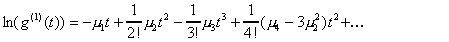where {mi} are the central moments of the distribution of interest P(G). The average gamma value is equal to the first moment, and its standard deviation is given by the square root of the second moment. The third moment theoretically describes the asymmetry of the distribution. The other coefficients are more difficult to interpret.

The coefficients {mi} may be easily found by solving the corresponding system of linear equations. Stabilization is achieved by reducing the number of unknown coefficients used to approximate ln(g(1)(t)). Generally, only the first two moments (and thus the average and the dispersion) can be reliably reconstructed. This provides very limited information for multi-component distributions or when dust is present.

#### A2. The Method of Histogram [10,11]:

This is the easiest method for extracting polydispersity information from PCS data. In the method of histogram, the number of unknown coefficients (histogram columns) in equation (6) is chosen to be small enough to make the solution stable. It is very important to divide the G axis logarithmically, otherwise the columns for low values of G must be made unnecessarily wide to provide stability for high values of G. It is also very important to use a non-negative least squares (NNLS) algorithm to restrict the solution to be non-negative. This information is very important and actually makes the algorithm usable by raising the number of histogram columns from 6-7 to 12-13 for the full reconstruction range [Gmin,Gmax].

The method of histogram has the following two drawbacks. Firstly, the maximum number of histogram columns which can be reliably reconstructed is not constant. Another drawback is that the logarithmic resolution is not optimal. Both the number of histogram columns and the optimal resolution law depend on the reconstruction range, the structure of the correlator in use, the noise in the experimental data and the distribution of interest itself. This dependence is not simple, and may be taken into account by using the singular value decomposition of the system (6).

#### B1. Methods using the Truncated Singular Value Decomposition [12,13,14]:

Singular Value Decomposition or simply SVD (see Section 3.1) is a powerful tool for solving unstable (ill-conditioned) systems of linear equations. Recall that as soon as the singular value decomposition of the system matrix A from (6) is computed, the solution is almost trivial x=VS-1UTg . The solution simply involves two multiplications by the orthogonal matrices UT and V and one multiplication by the diagonal matrix S-1=[1/s1,1/s2, ,1/sN]T. It has also been shown that the singular numbers, or more precisely the rate of their decay, defines the stability of the problem. The faster the decay, the closer the problem is to a singular one and the greater the uncertainty in the solution, thereby demanding more precise data to get the same information.

The advantage to using the singular value decomposition over other methods of solving the system of linear equations is that it allows us to easily remedy the solution to some extent by extracting only the stable or reliable information. This is done by replacing the matrix S-1 in the above equation with the matrix S+=[1/s1,1/s2,,1/sK,0,...,0]T where the singular numbers should be sorted in descending order, sK/s1>x and sK+1/s1<x . If we make a simple change of variables p=VTx, f=UTg and recall the properties of orthogonal matrices, the idea of remedying the problem with Singular Value Decomposition may be easily understood: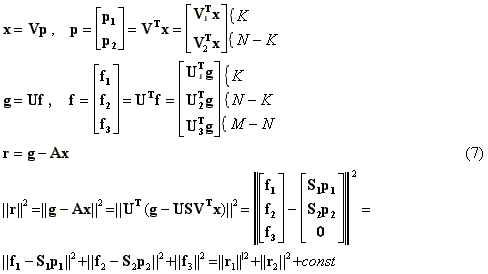where ||r|| is the residual norm, which is minimized in the method of least squares. If M>N, the last term is constant and cannot be minimized, no matter which method is chosen to solve the system of equations. In the method of truncated singular value decomposition, the second term ||r2||2 is not minimized either. Recalling the definition of the matrix S+ above, it leads to setting p2 to zero, ||r2||=||f2|| and ||r||2= ||r2||2+const. The hope is that sacrificing the residual norm to some extent, we can find a solution which is less sensitive to errors in the vector g. The idea may be more clearly presented if we use the property of orthogonal matrices ||x||=|| VTp|| and write the full expressions for the residual and the solution norms: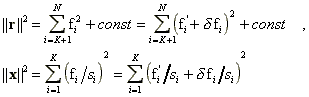where as before, f'=UTg' and df=UTdg denote the transformed exact data and the error vectors respectively. It can be seen that incrementing K leads to the reduction of the squared residual norm on the quantity fK2 and the enlargement of the squared solution norm on the quantity (pK/sK)2. Using functional analysis it may be shown that without the error term df, the coefficients {fi} decay to zero and thereby compensating for the influence of the small singular numbers. The errors {dfi}, divided by small singular numbers, introduce large, wild, components into the solution, making it totally inappropriate. The art of using the truncated singular value decomposition is to find a compromise (an appropriate K) between the residual and solution norms, keeping them both relatively small. Generally, if some freedom is allowed in the residual norm, there exist an infinite number of solutions possessing the same residual norm. It is important to note that of all these solutions, the solution computed by the truncated SVD possesses the smallest Euclidean norm.

The good news for users of truncated SVD is that the appropriate value for the component K can almost always be found. The bad news is that in our particular case its value is too small. Without using any other a priori information, this number is generally limited to 4 - 6 values which gives a very rough solution. Worse than that, if the distribution of interest is computed in a relatively wide range [Gmin,Gmax], it will have large oscillating or negative tails which have nothing to do with the true distribution. Additional information is necessary to make the method of truncated SVD useful. Different attempts have been made to impose auxiliary constraints on the solution, but, prior to the development of the unique algorithms implemented in DYNALS, no adequate solution suitable for commercial use was found. In these algorithms, the truncated singular value decomposition is combined with non-negativity constraints on the solution. This eliminates oscillation and negative tails and allows numerical analysis of the vector f so that the number K can be chosen automatically.

#### B2. Methods using Tikhonov's Regularization:

The most well known software program that uses Tikhonov's regularization method for processing experimental data in Photon Correlation Spectroscopy, is CONTIN [8,9]. Another, less known but good, example is the SIPP program described in .

In Tikhonov's method of regularization for the continuous case, the corresponding system of normal equations is used to find the unknown coefficients. The system is derived from the system of linear equations (6) by multiplying its left side by the matrix AT as Fx=b , where F=ATA and b=ATg . The exact solution of such a system minimizing the norm ||Fx-b|| is equivalent to the least squares solution of system (6), and is therefore unstable. To make it stable, the initial expression for the norm to be minimized is replaced as follows: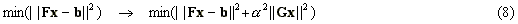where the term ||G||2, called the regularizer, defines the additional properties in favor of which we are ready to sacrifice the best possible fit. For example, if we would like to minimize the solution norm, we can set G=I, where I is the identity matrix. Most often, the matrix G encompasses a value associated with a 'smooth' solution. For example, this value may be the first or second derivative operator or its combination with the identity matrix. The coefficient a, called the regularization parameter, allows us to define the strength of the constraint on the solution. Very small numbers or zero do not introduce a constraint, and as a result the computed solution is unstable and has little in common with the actual solution. On the other hand, numbers that are too large pay little attention to the data, resulting in a solution that is smooth and nice but 'underfits' the data and hence extracts only part of the information available in the experimental data.

Technically, the method is implemented by using the modified system of normal equations devised as follows: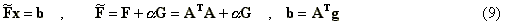Applying the singular value decomposition, it may be shown that using the solution norm as the regularizer (G=I) is equivalent to replacing the singular numbers with si'=(si2+a)1/2. Such substitution alleviates the problems associated with small si by damping large elements of S+ in accordance with the formula: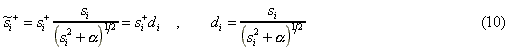Other regularizers provide different damping factors but employ a similar idea - they all decay smoothly towards zero as the corresponding index i increases. Alternatively, the solution computed by the truncated singular value decomposition may be associated with the damping factors computed as: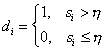where h is some threshold value. The proper choice for h is discussed in the following section devoted to the algorithms implemented in DYNALS.

In our case, there are two major difficulties associated with the method of regularization. The first one is related to the fact that the singular numbers decay very fast while the damping factors decay slowly. If a is chosen to damp all problematic singular numbers, it will partially damp the stable ones and, therefore, not all available information will be extracted from the data. On the other hand, if a is chosen to pass all valuable information, it will also allow small singular numbers to ruin the solution. The value in the middle will partially damp the stable information and partially pass the amplified noise (by dividing it into small singular numbers) and, as a result, the optimal solution is never found. A large number of published papers have been devoted to the optimal choice of the regularizer and the regularization parameter. Most, if not all of them, assume that the problem is linear, which in our case greatly underestimates the regularization parameter. The reason for such underestimation is that the system of equations is to be solved under the constraint of a non-negative solution. This constraint greatly improves the stability of the problem but causes the problem to be nonlinear (by the definition of a linear problem) and the corresponding, "optimal" a to become irrelevant.

### 3.4 Overview of the Algorithms Implemented in DYNALS

As mentioned previously, the algorithm implemented in DYNALS makes use of the singular value decomposition, but combines it effectively with the non-negativity constraint on the solution. To achieve this, the following function is minimized, while the target solution vector x is constrained to be non-negative: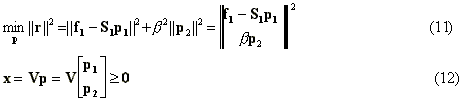where all the vectors and matrices are defined in equations (7) above. The expression (11) for minimization of the norm may seem to resemble the expression (8) minimized in the method of regularization (Section B2 above). However, in (11) different vectors, p1 and p2, are used in the two terms of the minimized function. The first vector, p1, is used to provide the minimal residual norm using only those stable components of the singular value decomposition corresponding to the large singular numbers. The second vector, p2, is used only to make the solution vector x non-negative. If the solution x, provided by unconstrained minimization of ||f1­S1p1||, is non-negative (or the constraint (12) is not applied), then nothing prevents p2 from being equal to zero and the solution is equivalent to the truncated singular value decomposition. Otherwise, the vector p2 is not zero and its components manifest additional information introduced by the non-negativity constraint. In other words, the vector p2 serves to give freedom to the vector p1, such that some tradeoff between the minimal truncated residual norm ||f1-S1p1|| and minimal truncated solution norm ||p2|| is reached. The tradeoff depends on the parameter b as follows: If b is too large, the second term ||p2|| is excessively constrained and does not provide enough freedom to the vector p1 (due to the non-negativity constraint) and the solution underfits the data. On the other hand, if b is too small, the vector p2 may take on wild values just to satisfy the constraint (12). The optimal value is found to be directly proportional to the accuracy of the experimental data, the maximal singular number and the norm ||f1||. The explanation of such proportionality is beyond the scope of this document.

Technically, the problem of minimizing (11) under the constraints (12) is solved by transforming the problem into the equivalent LDP (Least Distance Programming) problem, which is then solved by the method described in .

To implement the equations (7,11,12), we must divide the set of singular numbers into two subsets, the subset K "big" numbers and the subset N-K "small" numbers. If the input data were perfect, we might regard all the singular numbers as "big" so that K would be equal to N  Footnote3. When noise is present, this type of solution will be unstable. Therefore, the size of the first subset must depend on the accuracy of the experimental data. On the other hand, the relative influence of the random noise on the elements of f is also different. The vector f may be rewritten as f=f'+df=UTg'+UTdg . It can be shown that the elements of f' rapidly decay to zero while the standard deviation of the elements df remains the same (orthogonal transform of a random vector). It seems reasonable to minimize the residual norm using only those elements of f which significantly exceed the standard deviation of the elements df . With this choice, the size of the subset for "big" singular numbers depends not only on the noise in the experimental data, but on the distribution of interest itself. This is reasonable since a smaller number of singular value decomposition components are necessary to approximate a "smooth" distribution than to approximate a "sharp" one.

In DYNALS, the tail of the vector f=UTg is used to find sn (the standard deviation of the noise). After that, the noise level is set as 3sn and only those elements of f that are larger than this level are used for residual norm minimization. The actual implementation is a bit trickier when coping with the different complications caused by unusual noise statistics or non-random noise (systematic error), but the idea is the same.

### 3.5g(2)(t) to g(1)(t) Conversion or "Square Root" Trouble 

The first order correlation function g(1)(t) is used to compute the distribution of interest R(G) from the equation (3). This function is related to the normalized experimental data by the equation (2) which includes the experimental noise z(t). Due to the noise, the direct computation of g(1)(t) from g(2)(t) given by the following equation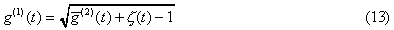is associated with certain complications. First of all, for large values of t, the expression under the square root sign may be negative. In this case, calculating g(1)(t) requires computing the square root of a negative number. The standard procedure used in photon-correlation spectroscopy uses the trick of setting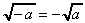; this is reasonable, but odd from a purely mathematical point of view. Actually, using complex numbers does not eliminate the problem's difficulty. The function g(1)(t) is real and the imaginary parts must be dropped for further processing. This is equivalent to setting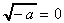which is worse than the standard rule and leads to larger systematic errors in the values of g(1)(t).

 shows that even when the value under the radical is non-negative, calculating g(1)(t) from (13) increases the random error (relative to g(2)(t)) and introduces a systematic error.  also proposes to compute the distribution of interest in two stages. In the first stage, the self-convolution of the distribution of interest R(G)* R(G) is computed from the function g(2)(t)­1. The smoothed estimation of g(2)(t) is then computed and used to compute the first order correlation function g(1)(t). Computer experiments have shown that this type of two stage processing provides better resolution. The option of two stage processing will be implemented in a future version of DYNALS.

In the meantime, weighting is used to alleviate the problems described above. Every row i of the matrix A in (6) and every element i of the data vector g are multiplied by the corresponding weight wi . The weights are set in accordance with  as: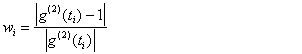providing good results (giving a random residual vector) in most cases.

## 4. Building your own Software for Processing PCS Data

The DYNALS processing capabilities are based on a set of well-defined processing APIs. The APIs are written in C in the object oriented manner and may be easily incorporated into another, proprietary interface. Please contact the authors for more details.

## 5. Footnotes

The order of footnotes corresponds to the sequence of their appearance in the document.
1. In some software programs this constant may be estimated separately as an independent parameter. For example, in CONTIN it is called the "dust term". In an ideal case, it must include everything which is constant in the data and leave the other components to be approximated by the distribution. In practice, it either underestimates or overestimates the true value, thereby sometimes distorting the distribution. It is better to extend the boundary corresponding to slow exponentials and let the optimization procedure decide what belongs where.
2. The fact that the best resolution is in the middle of the DYNALS default distribution domain, may be used to choose the optimal parameters of an experiment. For example, if the whole computed distribution of decay rates or diffusion coefficients is in the left half of the plot, try to reduce the sampling time. If it is in the right half, try to make it larger. If you chose the units as decay times or radii, just choose the other type of units.
3. The author does not discuss the problems related to the computer precision and discretization of equation (3). These problems, although theoretically interesting, are of minor concern, since they are automatically solved as soon as the noise in the experimental data is taken into account.## Appendix A.  Practical Exercises Using DYNALS

This appendix gives you step-by-step instructions which will help you to understand the problem and complexity of Particle Size Distribution Analysis in PCS. These exercises may be regarded as a tutorial that will provide you with the necessary skills, tips and hints for successful DYNALS operation. You will see how simple it is to solve this severe mathematical problem with DYNALS and how simple it is to check that you get the right solution.

All exercises are performed on synthetic data, which has two main advantages. Firstly, you know the true distribution in advance, which is very important when you are trying to understand the character of distortions caused by the stabilization procedure. Secondly, synthetic experimental data do not contain systematic error. Systematic error is generally very difficult to separate from data, and sometimes leads to the unpredictable distortion of results.

There is a variety of different synthetic data provided with DYNALS, along with a complete list describing the files. Several files, corresponding to different experimental noise levels, are provided for each distribution. It is supposed that the noise is normally distributed and uncorrelated between the channels. The last four numbers in the name of every file indicate the standard deviation of the error. For example, files 'xxxx0000' correspond to precise data, files 'xxxx0010' correspond to the standard deviation 0.001, etc. Files whose name begins with 'u', correspond to the uniform 100 channel correlator and a sampling time of 1 microsecond. Files whose name begins with 'l', correspond to the 50 channel logarithmic correlator and the same sampling time. You can look at the files to see how the channels are distributed.

The exercises may be performed using any distribution units (decay rates, decay times, diffusion coefficients or hydrodynamic radii), however we recommend using decay rates. The screen shots provided below correspond to decay rates in megahertz.

## A.1. Understanding the Term "ill-posed problem"

As written above, the problem of computing Particle Size Distribution from PCS data is an ill-posed mathematical problem. In our case, the term "ill-posed problem" designates a situation where there is an infinite number of absolutely different distributions R(G) that fit the experimental data g(2)(t) well enough (i.e. within the accuracy of experimental data). On the other hand, the solution which provides the best fit for experimental data is not always the one we would like to pick, as it may have nothing in common with the true distribution. Let us check this in practice.

In this exercise we use a wide, unimodal distribution. Load the file up1w0000.tdf corresponding to the accurate data and click the Start button. After the processing has finished, you will see the following distribution which is the true one.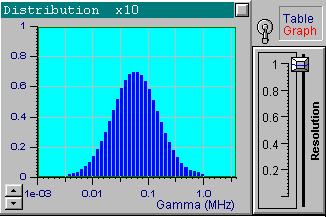Note that DYNALS sets the maximal possible resolution since the data are considered perfect. It does not mean that there is no stabilization; a minimal stabilization is used to compensate for finite computer arithmetic precision.

We will now choose another data set from the file up1w0010.tdf, which corresponds to the same distribution but includes noisy data. As indicated by the filename, the noise standard deviation for this file is 0.001. Click the Start button to receive the first in the following series of four pictures. These pictures correspond to the optimal resolution that is automatically set by DYNALS. The corresponding residuals are random, i.e., this distribution provides a good fit for experimental data. The position of resolution slider leaves you some freedom to experiment. By increasing the resolution slider value you can see that the shape of the distribution changes dramatically, while the residuals actually stay the same. The other three pictures provide examples corresponding to three different positions of the resolution slider. Each of these four distributions, along with an infinite number of the others corresponding to values of the resolution slider larger than the optimal one, provide a good fit to the data. Try using values that are less then optimal. You will see that the distribution computed using smaller resolution slider values does not provide a good fit.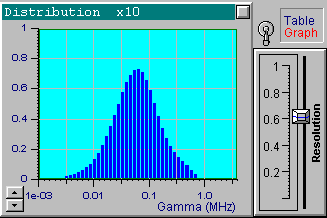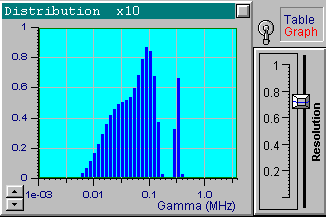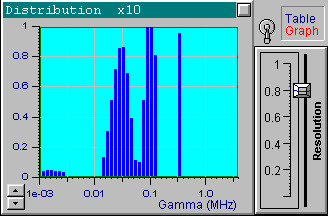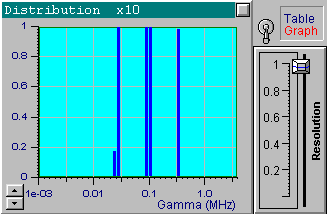Now load the file up1w0030.tdf, which corresponds to the same distribution but has more noise. After processing the data, you will see that the optimal resolution value is around 0.5. This value is lower than the one used for the previous file, which reflects the fact that the data is less accurate and more freedom is left for the solution. Try changing the position of the resolution slider to see a variety of the possible solutions.

## A.2. Understanding the Resolution and its Dependencies

If you measure a distribution of some physical quantity, it is natural to question the kind of resolution you should expect. In this context, the resolution may be defined as the minimal ratio in the positions of two separate distribution components, represented by two distribution peaks. In PCS, the distribution of interest is not measured directly, but is obtained as a result of the complex calculations necessary to resolve the integral equation (3). Consequently, the question of resolution in PCS is rather complicated and cannot be answered in two words. The resolution depends on several factors: the accuracy of experimental data, the correlator structure, the position of the distribution on the G axis (i.e. sampling time) and the shape of the distribution itself. In this section we will see how these four factors affect our ability to distinguish separated components.

### Dependence on the accuracy of experimental data:

It is intuitively clear that resolution must depend on the accuracy of experimental data. Let us check it in practice. For our experiments we will use a distribution that consists of two narrow peaks placed at G1=0.06MHz and G2 =0.18MHz with G2 / G1=3. The distribution is placed in the middle of the DYNALS default restoration range, which is the area of optimal resolution (we will check this later). The following four pictures show the results corresponding to the optimal resolution for the case without noise and the cases where the noise has the standard deviations 0.001, 0.003 and 0.01 (files up2n0000.dat, up2n0010.dat, up2n0030.dat, and up2n0100.dat respectively).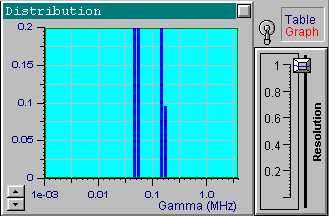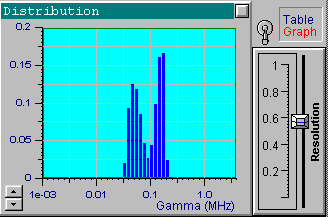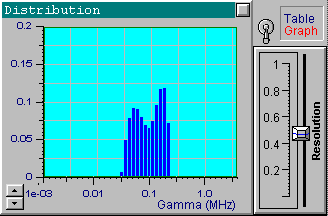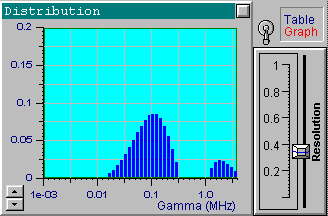It is clear that the resolution decreases as the noise increases. Note how the resolution slider reflects the accuracy of experimental data. You can see a small separate peak in the last picture (the most noisy data). This peak corresponds to very fast exponentials which do not actually exist. Its presence is explained by the large noise in the first one or two channels which is confused with very fast decaying components. There is nothing we can do with it, except perhaps to avoid fitting the first channels for very noisy data. Try it. It helps in this case. You can also try using the a priori information that the distribution of interest consists of narrow peaks. By setting the maximal value of the resolution slider, you will get the same sharp distribution shown in the first picture. Be careful with this approach though, for a relatively large noise and a multi-component distribution you may get incorrect peak positions.

### Dependence on the position of the distribution or sampling time:

This document states that resolution also depends on the position of a distribution on the G axis. To check this, let us take the same two modal, sharp distributions used in the previous section, and shift their position to the left and to the right, while maintaining the ratio of peak positions (using the files up2b0010.dat and up2c0010.dat ). The following two pictures reflect the results for the noise standard deviation 0.001, which is the same as for the right upper picture above.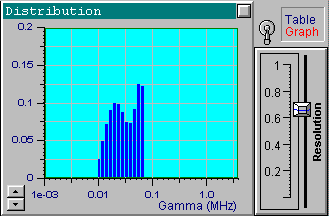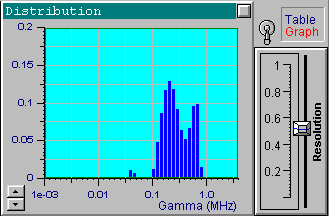As you can see, the peaks are less resolved than when located in the optimal resolution region. If the reconstruction region is defined automatically, this region is always located in the middle of the plot, which may serve as a good hint for a proper choice of the sampling time. If the decay rates or the diffusion coefficients are set as the reconstruction units, then reducing the sampling time corresponds to the distribution being shifted to the left (exponentials decay slower). Increasing the sampling time accelerates the decay and the distribution moves to the right. If the decay times or hydrodynamic radii are used, then the opposite is true.

### Dependence on the shape of the distribution:

It may not be obvious, but possible resolution of the distribution details depends on the shape of the distribution itself. Generally, the more complex the distribution, the less details it is possible to reconstruct. For example, we have seen that it is possible to resolve two peaks with G2 / G1=3. Using two such peaks, we will add another one which is rather far from the first two. We will then move the third peak towards the first two and observe what happens. For this experiment we will use data files corresponding to a 100 channel, non-uniform correlator. You can easily see the channels' location on the time axis by choosing the "time" units for the X axis of the data graph. The following four pictures correspond to the files np3a0010.dat, np3b0010.dat, np2c0010.dat, and np3d0010.dat respectively, processed with the default optimal resolution. We have also increased the number of distribution columns because of the larger possible restoration range covered by a non-uniform correlator.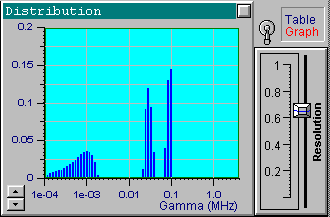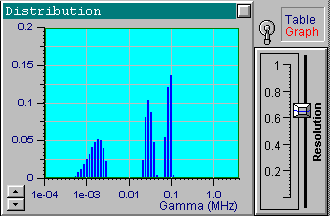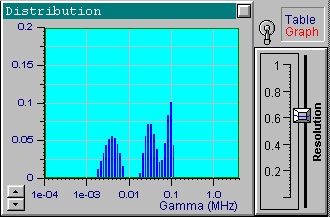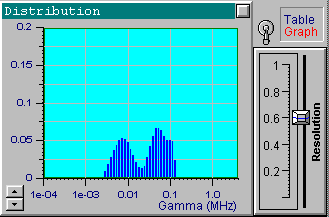It is obvious that the resolution depends on the distribution itself. The algorithm implemented in DYNALS makes a special analysis of experimental data and chooses the optimal (i.e. the maximal possible) resolution when no extra, a priori information is available.## Appendix B.  Tagged Data Format (TDF)

Tagged Data Format files (TDF files) are ASCII files similar to Windows INI files. These files consist of sections defined by the section name enclosed in brackets. Every section contains a specific kind of information that is further subdivided by tags. Every line of information begins with a tag identifying the information. An equals sign is used to separate each tag from its information. A typical TDF file containing PCS data looks like this:

[PCSPHYSPAR]
WAVELENGTH=  632.8
VISCOSITY=   1.0
SCATANGLE=   90.0
TEMPERATURE= 293.0
REFRINDEX=   1.33

[PCSDATA2]
1=   1.000000e-006 1.760349e+000
2=   2.000000e-006 1.596440e+000
3=   3.000000e-006 1.480216e+000
. . . . . . . . . . . . . . . .
99=  9.900000e-005 1.001706e+000
100= 1.000000e-004 1.002411e+000

The brackets are part of the syntax. The order of the sections and the tags inside the sections is arbitrary. The following section names are recognized by DYNALS :

[PCSDATA2] -- for the second order (intensity fluctuations) auto-correlation function measured under the homodyne scheme. The section's tags define the numbers of corresponding correlator channels. The information field consists of two numbers: time delay (in seconds) corresponding to the particular channel and the normalized correlation function value in that channel.

[PCSPHYSPAR] -- physical parameters of the experiment where the data were acquired. The following tags are recognized in this section:

• WAVELENGTH - wavelength of the scattered light
• VISCOSITY - viscosity in cP
• SCATANGLE - scattering angle in degrees
• TEMPERATURE - temperature in degrees Kelvin
• REFRINDEX - refraction index

## References

1. Gulari Es., Gulari Er., Tsunasima Y. and Chu B., J. Chem. Phys. 70.80, 3965­3972 (1979).
2. Tikhonov A.V. and Arsenin V.Y., Solution Ill-posed problems, Winston & Sons, (1977).
3. Koppel D.E., J.Chem.Phys. 57, 4814, (1972).
4. Pusey P.N., Koppel D.E., at all, Biochemistry 13, 952, (1974).
5. Ostrowosky N. and Sornett D. - in Photon Correlation Techniques in Fluid Mechanics, Springer, Berlin, 286, (1983).
6. Chu B., Correlation Function Profile Analysis in Laser Light Scattering, in "The Application of Laser Light Scattering to the Study of Biological Motion", NATO ASI Marateo, (June 1982).
7. J.Chem.Soc. Faraday Trans. 2.75, 141 (1979).
8. Provencher S., Comput.Phys.Commun., 27, 213-227 (1982).
9. Provencher S., Comput.Phys.Commun., 27, 229-242 (1982).
10. Gulari Es., Gulari Er., Tsunashima Y., J.Chem.Phys., 70, 3965.
11. Chu B., Gulari Es., Gulari Er., Phys.Scr., 19, 476 (1979).
12. Bertero M., Boccacci P., Pike E.R., Proc. R. Soc. Lond. Ser.A, v383, 51 (1982).
13. Bertero M., Boccacci P., Pike E.R., Proc. R. Soc. Lond. Ser.A, v398, 23 (1985).
14. Bertero M., Boccacci P., Pike E.R., Proc. R. Soc. Lond. Ser.A, v383, 15-29 (1983).
15. Danovich G., Serduk I., - in Photon Correlation Techniques in Fluid Mechanics, Springer, Berlin, 315 (1983).
16. Lowson C.L. and Hanson R.J., Solving Least Squares Problems, Englewood Cliffs,, NJ: Prentice Hall, (1974).
17. Goldin A.A., Opt.Spectrosk. 71, 485-489 (September 1991).
18. Provencher S., Makromol.Chem. 180, 201 (1979).